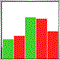# Master Candle's Trendlines until it's broken540

Hello all,
i am writing a "Master Candle" indicator (on H1).

Master candles are highlighted, but now, i need to draw high and low trend lines until the price break these high or low.
Then the trendline are stopped to be drawn on the (H1) candle breakout.
How can i do that  please ?

Here is my code :
```for(int i_H1=0;i_H1<MC_H1_Range_To_Process;i_H1++)
{
string tname=NULL;

datetime t_Start=0;
datetime t_End=0;
datetime t_Master_Start=0;
datetime t_Master_End=0;

double high=0;
double low=0;
double master_high=0;
double master_low=0;

if(TLR_Button_MC_H1_Range_State==true)
{
// --- HL rectangles
tname=TimeToString(iTime(NULL,PERIOD_H1,i_H1+1));

t_Start=iTime(NULL,PERIOD_H1,i_H1+1);
t_End=iTime(NULL,PERIOD_H1,i_H1+0);
t_Master_Start=iTime(NULL,PERIOD_H1,i_H1+2);
t_Master_End=iTime(NULL,PERIOD_H1,i_H1+1);

high=iHigh(NULL,PERIOD_H1,i_H1+1);
low=iLow(NULL,PERIOD_H1,i_H1+1);

master_high=iHigh(NULL,PERIOD_H1,i_H1+2);
master_low=iLow(NULL,PERIOD_H1,i_H1+2);

RectangleCreate(0,                             // chart's ID
"TLR_MC_H1_Range_H1_HL"+tname, // rectangle name
0,                             // subwindow index
t_Start,                       // first point time
high,                          // first point price
t_End,                         // second point time
low,                           // second point price
Levels_H1_Dark_clr,            // rectangle color
0,                             // style of rectangle lines
0,                             // width of rectangle lines
false,                         // filling rectangle with color
false,                         // in the background
false,                         // highlight to move
true,                          // hidden in the object list
0);

// --- HL master candles
if(master_high>=high && master_low<=low)
{
RectangleCreate(0,                             // chart's ID
"TLR_MC_H1_Master_H1_HL"+tname,// rectangle name
0,                             // subwindow index
t_Master_Start,                // first point time
master_high,                   // first point price
t_Master_End,                  // second point time
master_low,                    // second point price
Levels_H1_Dark_clr,            // rectangle color
0,                             // style of rectangle lines
0,                             // width of rectangle lines
true,                          // filling rectangle with color
false,                         // in the background
false,                         // highlight to move
true,                          // hidden in the object list
0);
}
}

if(TLR_Button_MC_H1_Range_State==false)
{
tname=NULL;
t_Start=0;
t_End=0;

high=0;
low=0;
// ...etc
}
}```
Regards.540

here is a picture of what I have now :
- (H1) ranges and master ranges are already calculated.
- the white trend lines are made by hand.

How can i draw the trend lines and stop drawing it when the price have broken the high OR the low ?

regards.